## Example Questions

← Previous 1 3

### Example Question #1 : How To Divide Integers

A car averages 29 miles per gallon.  If gas costs $3.75 per gallon how much money would need to be spent on gas to travel 1464.5 miles? Possible Answers:$189.38

$5491.88$50.5

$108.75 Correct answer:$189.38

Explanation:

The question is asking for the amount of money that would need to be spent on gas.  Using the value given for the miles per gallon of the car and the amount of miles traveled it is possible to determine the gallons of fuel that will be required.  This will be set up as 1464.5 miles travelled divided by the 29 miles per gallon that the car gets.  This leaves us with 50.5 gallons of fuel used.  From this point we can multiply the amount of fuel used, 50.5 gallons, by the price of fuel per gallon, $3.75, to obtain the amount of money that will spent on fuel,$189.38.

### Example Question #1722 : Act Math

Connie's car gets 35 miles per gallon of gas.  How much gas will she need to take a 525 mile trip?  Round to the nearest gallon.

17

20

25

15

23

15

Explanation:

This becomes a division problem: 525 miles ÷ 35 miles per gallon = 15 gallons

### Example Question #3 : How To Divide Integers

A father buys a bag of marbles.  He divides the marbles among his 5 children, who receive 24 marbles each. If there had been 6 children, how many marbles would each one get?

22

24

20

18

20

Explanation:

There were a total of 120 marbles to begin with, since 5 * 24 = 120.

If the marbles are split between 6 children, then each child gets 120/6 = 20 marbles each.

### Example Question #4 : How To Divide Integers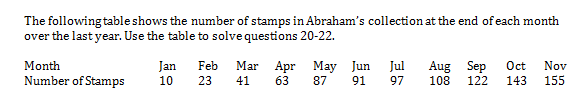At the end of December, Abraham adds the average number of stamps he has been collecting over the year to his album, how many stamps will he have at the end of December?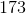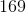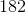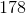Explanation:

In 11 months, Abraham has collected 155 stamps, or an average of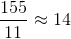stamps per month. At the end of December, he should have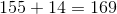stamps.

### Example Question #1 : How To Subtract Integers

On an airline flight of 250 people there is a choice of pasta, salad, or pizza for dinner. If 113 passengers choose pasta and 30% choose salad, how many passengers choose pizza?

62

53

100

75

62

Explanation:

30% of 250 is 75. Therefore, 250 – 75 – 113 = 62

### Example Question #2 : How To Subtract Integers

At a store, a t-shirt costs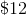. If Shane boughtt-shirts and shoes from the store for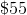, how much were the shoes?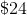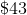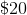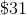Explanation:

If one shirt costs, then two shirts must cost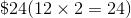. The difference of the total and this number is the cost of the the shoes.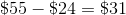### Example Question #3 : How To Subtract Integers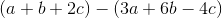is equivalent to which of the following?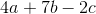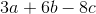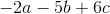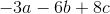Explanation:

To answer this question, we must remember how to distribute a negative when we are subtracting an entire expression.

To distribute a negative, you change the sign of everything within the expression being subtracted from positive to negative or negative to positive. You then add the resulting expression. So, for this data: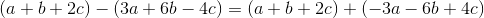We can then add the like expressions normally (combinewith,with, andwith). Therefore, for this data: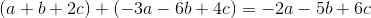Therefore, our answer is.

### Example Question #1 : How To Multiply Integers

A hockey team wants to purchase uniforms for its 26 players, 2 of which are goalies.  It costs $40 for a one-time designing fee. It costs$24 for goalie jerseys and $20 for all other jerseys. How much does it cost to purchase all of the uniforms? Possible Answers:$520

$568$608

$528$664

$568 Explanation: There are 24 normal players and 2 goalies. Thus we can represent the sum in the equation 24($20) + 2($24) +$40 = $568. ### Example Question #2 : How To Multiply Integers Sam is paid$15 per hour for laying bricks. If he lays 3,000 bricks at a rate of 5 bricks per minute, how much will he be paid?

$9,000$200
$150$300
$1,500 Correct answer:$150
Explanation:

First we need to convert some units. If Sam lays 5 bricks per minute, then multiply that figure by 60 to find that he lays 300 bricks per hour. 3,000 bricks divided by 300 bricks/hour means he worked for 10 hours. Therefore, when we mutiply $15/hour by 10 hours, we see he makes$150.

### Example Question #3 : How To Multiply Integers

Joey is beginning to invest in the stock market. He purchased 150 shares of a cell phone company.  The company pays a dividend of $2.55 per share. What is his total dividend? Possible Answers:$451.75

$280.25$301.25

$255.00$382.50